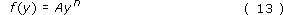Describing motion along a line

Start this free course now. Just create an account and sign in. Enrol and complete the course for a free statement of participation or digital badge if available.

Free course

# Functions and the function notation

In Figure 25, the position x of the car depends on the time t. The graph associates a particular value of x with each value of t over the plotted range. In other circumstances we might know an equation that associates a value of x with each value of t, as in the case of the equation x = At + B that we discussed in Section 3. You can invent countless other ways in which x depends on t: for instance x = At2 + B or x = At + Bt﻿2. In all such cases we describe the dependence of x on t by saying that x is a function of t. This terminology is widely used and is certainly not restricted to x and t. In the example of non-uniform motion we have just been discussing, the instantaneous velocity v﻿x is a function of time and so is the instantaneous acceleration a﻿x.

Generally, if the value of a quantity f is determined by the value of another quantity y, then we say that f is a function of y and we use the special notation f(y) to emphasise this relationship. Although we have only just defined what we mean by a function we have already been using this notation, as in v﻿x﻿(﻿t﻿) and a﻿x﻿(﻿t﻿), for some time.

This function notation has two great merits:

1. Writing f﻿(﻿y﻿), provides a clear visual reminder that f depends on y in a well-defined way. If we happen to know the equation that relates f to y, say f = y﻿2, then we can show this explicitly by writing f﻿(﻿y﻿) = y﻿2.

2. If we want to indicate the value of f that corresponds to a particular value of y, it is easy to do so. For example, the value of f﻿(﻿y﻿) at y = 2 can be written f﻿(﻿2﻿). We call f﻿(﻿2﻿) the function value at y = 2. Of course, in order to be able to write f﻿(﻿2﻿) as a number we would have to know the explicit form of f﻿(﻿y﻿). For example, if f﻿(﻿y﻿) = y﻿2, then we can say f﻿(﻿2﻿) = 2﻿2 = 2 × 2 = 4.

The only serious disadvantage of the function notation is that you may confuse f(y) with f × y. Be careful! If f is a function, then f﻿(﻿y﻿) means f is a function of y; it does not mean f × y.

Some functions arise repeatedly and are given special names so that they can be easily identified. You will be familiar with some of these names, even if you are not yet fully familiar with the functions they describe. For instance, if you look at a scientific calculator (see Figure 31 for example) you will sometimes find that there are keys labelled sin, cos, log and ex; each of these is the name of an important function that you will meet later. The corresponding calculator keys are actually called 'function keys'. Electronic calculators are constructed in such a way that when you key in a number and press a function key, the calculator works out the corresponding function value and displays it. If the value you keyed in is not within the acceptable range of input values for the function you selected (usually called the domain of the function), then the calculator will probably display an error message such as 'err'.Figure 31: A calculator has in-built routines for evaluating basic functions such as x﻿2, sin﻿(﻿x﻿), cos﻿(﻿x﻿), log﻿(﻿x﻿), etc. These are activated by pressing the function keys

One particularly simple class of functions consists of functions of the formwhere A is a constant and n is a positive whole number, such as 0, 1, 2, 3, …. Functions of this kind include squares f﻿(﻿y﻿) = y﻿2, and cubes f﻿(﻿y﻿) = y﻿3, which correspond to A = 1 with n = 2 and n = 3, respectively. The function that arises when n = 0 is especially noteworthy since, by convention, y0 = 1, so f﻿(﻿y﻿) = A in this case, a constant. Thus, even a simple constant is just a special kind of function. In the case of a constant function each value of y is associated with the same value of f﻿(﻿y﻿), as in the case of the velocity-time graph of a uniformly moving object.

Next in complexity are sums of squares, cubes, etc. These functions are called polynomial functions and include the following special cases (where A, B, C and D are constants):

 linear functions of the form f﻿(﻿y﻿) = Ay + B quadratic functions of the form f﻿(﻿y﻿) = Ay﻿2 + By + C cubic functions of the form f﻿(﻿y﻿) = Ay﻿3 + By﻿2 + Cy + D.

In the case of uniform motion (described in Section 3), the position-time graph is a straight line described by an equation of the form x = At + B. It should now be clear that another way of describing this relationship is to say that the position is a linear function of the time, x﻿(﻿t﻿) = At + B. In a similar way, the non-uniform motion of a test vehicle in the NASA drop-shaft described at the beginning of this course can be described by a quadratic function x(t) = At﻿2 + Bt + C, and many other forms of one-dimensional motion can be described, at least approximately, by suitably chosen polynomial functions.

S207_2